Estimation by Generalized Method of Moments

Overview

Generalized Method of Moments is a class of estimators, or an estimation rule, that includes OLS, GLS and IV estimators as members.  The approach is based on the idea that the population central moments are known functions of unknown parameters of interest.  The population moments can be estimated by the sample central moments.  In turn, the sample moments are then used in place of the population moments in the known system of equations.

1. Except when the random variable being modeled is a member of the exponential family, the method of moment estimators are not efficient.

2. The method of moments is robust to the specification of the data generating process.  The sample mean is an estimator for the population mean, the sample variance is an estimator for the population variance, provided they both exist.

Some Examples of the Method of Moments

x1, ... ,xn are independent random variables. Take the functions g1, g2, ... , gn and look at the new random variables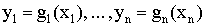then the y's are also independent. If all the g are the same, then the y are iid.

Now suppose x1, x2, ... are iid. Fix k a positive integer. Then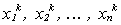are iid and by the weak law of large numbers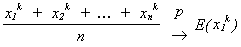which are the kth moments about the origin.

Define mk = Exk as the kth moment of x so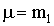and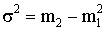Suppose you wish to estimate some parameter,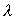.

We know mk = E(xk) for k = 1, 2, ... and suppose thatis some function of the central moments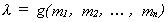and g is continuous.

The sample moment is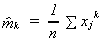Idea: If n, the sample size, is large then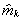should be close to mk for k = 1, 2, ... , N so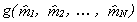should be close to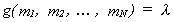.

Example:

The mean and variance as functions of the first two central moments.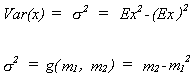The method of moments estimator for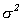is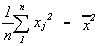, the second sample moment minus the square of the first sample moment.

Example:

The uniform distribution.

Consider X, a continuous random variable distributed as the uniform distribution on the interval [a, b]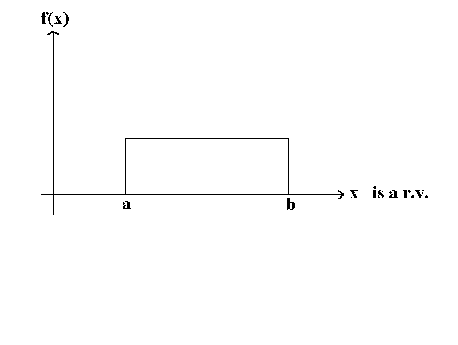We wish to estimate a and b, the two parameters that characterize the uniform distribution. From experience we know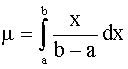and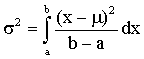from which we can find the population moments in terms of the parameters of the distribution.  We can then equate the sample moments to the population moments to solve for the unknown parameters of interest.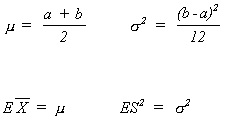Suppose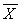= 5 and S2 = 2.5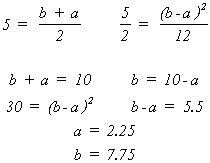Example:

The inverse Gaussian (Wald) Distribution is used to model elapsed times, e.g, time to failure of a part in a machine.  The shape and location of the density are determined by two parameters, μ and λ. The density is given by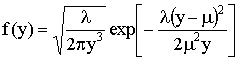The mean is μ and the variance is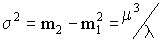.

A. The efficient maximum likelihood estimators of the two unknown parameters are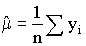and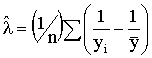B.  The sample mean and sample variance can also be used to estimate the parameters of the distribution as follows: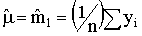and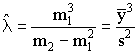This follows from the population variance expressed in terms of the central moments.

Example:

The Gamma Distribution has two determinative parameters, P and λ . The density is given by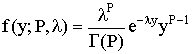The Gamma Distribution has as special cases the Chi-square and the Exponential distributions.

The log likelihood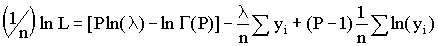The mean, m1, and variance, m2-m12, are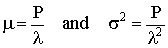from which we can see that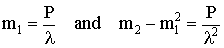and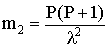.  One could estimate m1 and m2 by the respective sample moments.

Additionally, differentiating the likelihood function with respect to P yields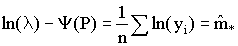From Poirier(1995) the moment generating function for the Gamma is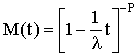One can differentiate M(t) with respect t and evaluate the result at t=0 to derive an expression for the rth central moment as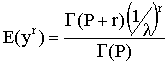setting r = -1 suggests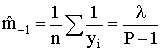We now have four moments and two unknown parameters: m1, m2, m*, m-1 and P, λ.

For Greene's income data the results are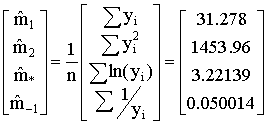Any pair of the sample moments can be used to construct estimates of the two unknowns: four things taken two at a time yields six possible pairs of estimates.  The maximum likelihood estimates are based on m1 and m*.

Generalized Method of Moments

Consider the garden variety regression model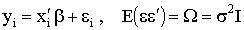(1)

We always assume the orthogonality condition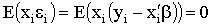The independent variables and the error term should be independent of one another.  There are k such equations, which are actually moment equations.  The sample analog of this is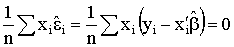This set of coefficients that satisfy these sample moments happen to be the least squares estimates.  We can show the same kind of results for the IV estimator.

Suppose we know that the orthogonality condition is violated for our model, but we have another set of variables, call them Z, for which the orthogonality condition holds, at least in the limit.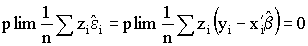When we have more instruments than unknown coefficients the model is said to over-identified.  The over-identification problem is resolved by solving the following sample moment functions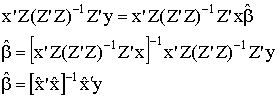(2)

Properties of the GMM estimator

Assumption 1 - The empirical moments converge in probability to their expectation.

Assumption 2 - For any n ≥ k, if θ1 and θ2 are two different parameter vectors, then there exist data sets such that mn1) ≠ mn2) holds for the empirical moments.

A2 ==>  1. The number of moment conditions is at least as large as the number of unknown parameters.
2. Suppose there are K unknown parameters of the distribution and L moment functions.  The matrix of first derivatives of the moment functions has full row rank.
3. Any parameter vector that satisfies the population moment condition is unique.

Assumption 3 - If the empirical moments have a finite asymptotic covariance matrix then they converge in distirbution to the normal distribution.

Theorem  Under the three assumptions the method of moments estimator converges in probability to the unknown parameter.  That is, the GMM estimator is consistent.  Also, the GMM estimator is asymptotically distirbuted as a normal random variable.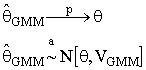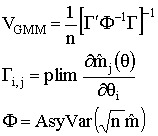The theorem allows us to implement, say, an asymptotic t-test or the Wald, Likelihood Ratio and Lagrange Multiplier tests.

The GMM estimator that we have been using is the solution to a criterion function, much like OLS and GLS are the solutions to a criterion function in which the sum of squared errors are minimized.  The GMM criterion function is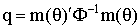Evaluate the three functions at the sample data and multiply by the sample size to get a χ2 random variable with L-K degrees of freedom.  L is the number of moment functions and K is the number of unknown parameters.  This test statistic can be used to test the validity of the overidentifying restrictions, and by extension also tests the validity of the underlying model.  In the above example using the Gamma distribution there were two extra moment equations, resulting in six pairs of estimates of the two unknowns.  This specification test can be used to decide whether the six sets of estimates are different from one another.  If they are statistically idfferent form one another then we conclude that the data were not generated by a Gamma.  As another example, in the IV case we often have L>K instruments, resulting in too many orthogonality conditions. If the test statistic described here is large then we conclude that some of the variables used instruments do not qualify as instruments and should have been included as regressors.

The same test statistic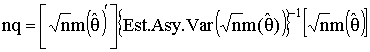can be evaluated at restricted and unrestricted versions of θ to construct a hypothesis about the parameter vector.

Another interpretation:

Suppose that in (1), above, Ω is not scalar diagonal.  Then it is possible to reinterpret the IV estimator in (2) in an alternative light.  Namely, we choose Z as the triangular square root of Ω-1, then proceed as with the IV estimator.  In the cases of heteroscedasticity and serial correlation we usually know enough about Ω to estimate it consistently.  Our conclusion is that the method of moments estimator is also heteroscasticity and autocorrelation consistent estimator.

An Example Specific to Economics

Life Cycle Consumption

This example is representative of a whole class of dynamic optimization problems. Suppose that the representative consumer has utility function over consumption each period and tries to maximize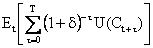subject to the wealth constraint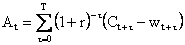where we have the following definitions

Et = expectation given the information available at time t

δ = rate of subjective tie preference of the consumer

r = fixed real rate of interest

T = length of economic life

Ct = consumption at time t

wt = earnings at time t

At = assets at time t

This model implies and Euler equation of the form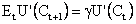Where U' is the marginal utility of consumption andThe Euler equation can be rewritten as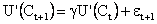where the error term εt+1 represents the divergence of discounted lagged marginal utility of consumption from its value today.  The error term has mean zero and is serially uncorrelated.  If δ = r, the consumer's rate of time preference is equal to the real rate of interest, then marginal utility would be a constant except for new information arriving between t and t+1.  Hence, the error or innovation to marginal utility is uncorrelated with information arriving on or before period t, this is in the nature of an orthogonality condition.  Let information arriving at time t be represented by Zt.  Then orthogonality implies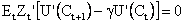Note that this is in the spirit of a first order condition from an instrumental variables estimator as we saw it earlier in the notes of this section.  Now assume that the utility function is quadratic in consumption, so that marginal utility is linear in consumption.  Accordingly we can write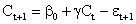Following the argument of earlier scholars like Duesenberry or Friedman we might include Ct or Income, y t , or their lags as instruments.  For the sake of the argument let us construct the instrument matrix as

Zt = [ 1 Ct yt ]

The test statistic that new instrument is a correctly excluded exogenous variable is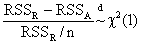where RSSR is the sum of squared residuals from the regression of Ct+1 on Ct and a constant, and RSSA is the residual sum of squares from the regression of the residuals from the restricted regression on income (yt).

The value of GMM is seen in this example when utility is not quadratic, in which case we could return to the orthogonality condition and estimate.

Robert Hall, "Stochastic Implications of the Life Cycle - Permanent Income Hypothesis: Theory and Evidence" Journal of Political Economy, Vol 86, 1978, Pp 971-987.

 Permanent Income, Current Income, and Consumption JOHN Y. CAMPBELL Harvard University - Department of Economics; National Bureau of Economic Research (NBER) N. GREGORY MANKIW Harvard University - Department of Economics; National Bureau of Economic Research (NBER) January 1991 NBER Working Paper No. W2436